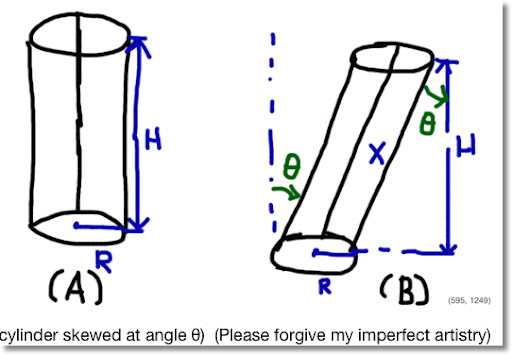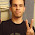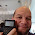## Wednesday, May 23, 2012

### Right Cylinders and Skewed Cylinders

Blog Entry # 103: One of my favorite things to do in mathematics is to find areas and volumes of unusual shapes.Volume of a Right Cylinder (Figure A)

V = π R^2 H

H = height

Volume of a Skewed Cylinder at Angle θ (Figure B)

H resembles the measure from the base to the top of the skewed cylinder. However, to calculate the volume, we will need the length X, not H.

Using trigonometry:

cos θ = H / X

implies that

X = H / (cos θ)

The volume of the skewed cylinder is:

V = π R^2 H sec θ

Until next time, Eddie

1.I think cos θ = X/H should be cos θ = H/X, you know cos θ = adj./hyp.

2.Thanks Mike. The correction has been made.

3.Thanks form this information.Mensuration is a very important topic of mathematics as it is very much related to our daily life like area and volume of different solid figures or area of plot,length and breadth of different object.It is interlinked with many real life problems.
Box and Whisker Plot

4.This doesn't seem right. They should have the same volume.

If you were to set up an integral, it would be best to slice vertically into "infinitesimally thin disks" then integrate vertically and the y axis which would just give Area of base (circle) times height (H)

Cavalieri's principle is a similar concept
http://en.wikipedia.org/wiki/Cavalieri%27s_principle

Lastly.... the reference section of my math textbook gives the equation for a generic (oddly shaped) cyclinder as being like that of a regular cylinder, Area of base x height

5.I believe what you calculated here was the volume of a regular (right) cylinder of base (pi)R^2 and height X (represented in terms of h)

Not skewed, but tilted. (which then has to be elongated so that the length measured along the y axis will be H)

I'm wondering if you can show prove this to yourself using a jacobian as well...

6.OK well I sort of was able to do a Jacobian. I figured that since the base is going to be the same, I'll just look at a skewed rectangle in the x-y plane

the Jacobian transform was 1, so no change in volume

After doing this, I realized how unnecessary it was, it's the same as that age old picture of proving that a parallelogram's area is the same as the area of a rectangle (by bringing that triangle from one side to the other)
like this: http://image.wistatutor.com/content/feed/tvcs/8sqng8v9zyt0tzo5c84.jpg

### Retro Review and Comparison: TI-82 Advanced

Retro Review and Comparison: TI-82 Advanced I can officially say that I have a French calculator.   Quick Facts: Models:  TI-82 Advanced Co...